SYLLABUS  Previous: 3.2 An engineer's formulation  Up: 3 FINITE ELEMENT METHOD  Next: 3.4 Implementation and solution

## 3.3 Numerical quadrature

Slide : [ Formula - Table - Tunable || VIDEO login]

Unless the coefficients of the differential equation (here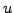and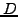) are singular and require a special treatment somewhere in the solution domain, the precision of the quadrature depends mainly on the choice of the basis and test functions: it is indeed sufficient to simply preserve the convergence rate that is guaranteed by the FEM discretization. Writing the numerical quadrature as a truncated sum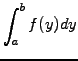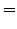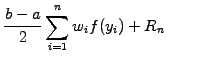(1)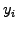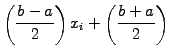(2)

table 3.3.0#tab.1 shows how a judicious choice of the abscissas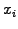and weights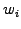yields an approximation of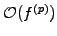that integrates exactly polynomials with a degree up to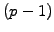.

Table 3.3.0#tab.1: Quadrature abscissas and weights for the interval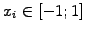quadrature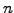terms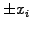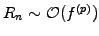mid-point 2 0 1 p=2 trapezoidal 2 1 1 p=2 Gaussian 2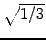1 p=4 Gaussian 3 0 8/9 p=6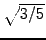5/9 Gaussian 4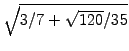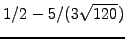p=8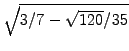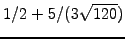For example, a superposition of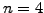terms (where the integrand is evaluated on the specified location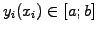and weighted by the factor) is required using a Gaussian quadrature, when four evaluations of the integrand in every mesh interval are used to compute exact overlap integrals involving the product of (two) cubic test and basis functions. In the case of linear roof-top functions, the mid-point and trapezoidal rules are both equally precise and require only one evaluation per interval; although this is slightly more expensive with one extra evaluation per interval, both rules can nicely be combined into a so-called tunable integration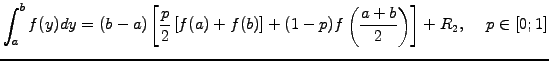(3)

As will become clear below, this allows to gradually modify the piecewise linear FEM discretization (obtained with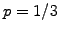) into either an equivalent FD discretization (with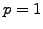) or a piecewise constant FEM approximation (with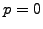). Apart from the academic interest, this feature can be used to change the slope of the numerical convergence and even to stabilize an approximation that is marginally unstable.

Armed with a variety of quadrature rules, it is now possible to implement the linear FEM scheme sketched in sect.3.2 using a tunable integration (3.3#eq.3) to evaluate the matrix elements in (3.2#eq.5). Since roof-top functions reach only as far as their nearest neighbors, all the matrix elements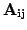with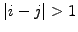vanish, except for the overlap at the boundaries. Choosing a sequential numbering of the unknowns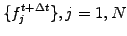, this results in a tri-diagonal matrix, with two extra elements in the upper-right and lower left corner taking care of the periodicity. It is a good exercise for the reader to verify that all the matrix elements can be written in terms of the integrals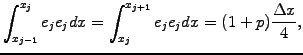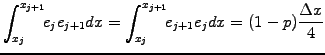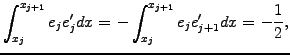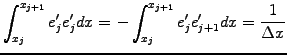provided that the advection and diffusion coefficients are constant across the intervals as will be assumed here for pedagogical reasons. In a real code constructed for an inhomogeneous medium, each formula is replaced byfunction calls returning the value of the integrand (where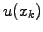,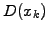are evaluated on quadrature points) and the weighted sum (3.3#eq.1) is simply accumulated in the corresponding matrix element.

SYLLABUS  Previous: 3.2 An engineer's formulation  Up: 3 FINITE ELEMENT METHOD  Next: 3.4 Implementation and solution